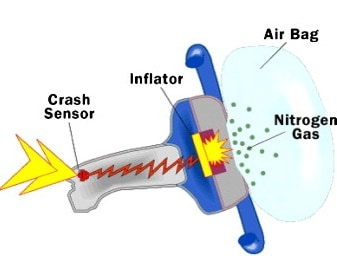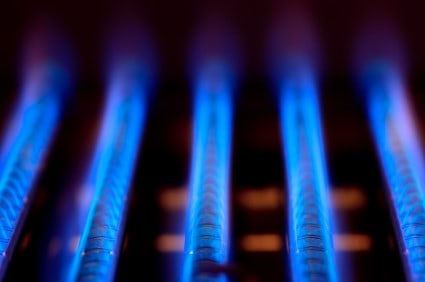## General Chemistry

Sometimes the amounts of gases need to be calculated based on chemical reactions. These calculations combine the ideal gas laws and the same principles we learn in stoichiometric calculations. The only difference for most problems is going to be that the quantities are given in mass or volume together with the pressure of the gas.

Remember that the link between any reactants and products in a chemical reaction is their molar ratio, so you need to always calculate the moles for solving these problems.

For example,

Sodium azide is used in automobile airbags as a source of nitrogen gas to rapidly inflate the bags upon a hit. If the volume of the airbag is 7.95 L, what mass of NaN3 is required to produce enough nitrogen to fill it at 23.0 °C and 1.20 atm?

2NaN3(s)  →  2Na(s)  +  3N2(g)The plan for solving this problem is to find the moles of nitrogen, use that to determine the moles of sodium azide based on the stoichiometric ratio, and finally convert the moles of NaNto the mass that is needed for producing the given amount of nitrogen gas.

${\rm{n}}\;{\rm{ = }}\;\frac{{{\rm{PV}}}}{{{\rm{RT}}}}$

${\rm{n}}\;{\rm{ = }}\;\frac{{{\rm{1}}{\rm{.20 }}\cancel{{{\rm{atm}}}}{\rm{ 7}}{\rm{.95 }}\cancel{{\rm{L}}}}}{{{\rm{0}}{\rm{.08206}}\;\cancel{{\rm{L}}}\;\cancel{{{\rm{atm}}}}\;\cancel{{{{\rm{K}}^{{\rm{ – 1}}}}}}\;{\rm{mo}}{{\rm{l}}^{{\rm{ – 1}}\;}}{\rm{296}}\;\cancel{{\rm{K}}}}}\;{\rm{ = }}\;{\rm{0}}{\rm{.393}}\;{\rm{mol}}$

${\rm{n(Na}}{{\rm{N}}_{\rm{3}}}{\rm{)}}\;{\rm{ = }}\;{\rm{0}}{\rm{.393}}\;{\rm{mol}}\;{{\rm{N}}_{\rm{2}}}\;{\rm{ \times }}\;\frac{{{\rm{2}}\;{\rm{mol}}\;{\rm{Na}}{{\rm{N}}_{\rm{3}}}}}{{{\rm{3}}\;{\rm{mol}}\;{{\rm{N}}_{\rm{2}}}}}\;{\rm{ = }}\;{\rm{0}}{\rm{.262}}\;{\rm{mol}}$

Check Also

#### Practice

1.

Oxygen can be prepared in the laboratory by decomposing potassium chlorate, KClO3 according to the following reaction:

2KClO3(s) → 2KCl(s) + 3O2(g).

How many liters of oxygen can be produced from 4.80 g KClO3, if the reaction is carried out at standard conditions?

This content is available to registered users only. Click here to Register! By joining Chemistry Steps, you will gain instant access to the answers and solutions for all the Practice Problems and the powerful set of General Chemistry 1 and 2 Summary Study Guides.
Solution
This content is available to registered users only. Click here to Register! By joining Chemistry Steps, you will gain instant access to the answers and solutions for all the Practice Problems and the powerful set of General Chemistry 1 and 2 Summary Study Guides.
2.

Sodium azide is used in automobile air bags as a source of nitrogen gas to rapidly inflate the bags upon a hit. If the volume of the airbag is 7.95 L, what mass of NaN3 is required to produce enough nitrogen to fill it at 23.0 °C and 1.20 atm?

2NaN3(s)  →  2Na(s)  +  3N2(g)

This content is available to registered users only. Click here to Register! By joining Chemistry Steps, you will gain instant access to the answers and solutions for all the Practice Problems and the powerful set of General Chemistry 1 and 2 Summary Study Guides.
Solution
This content is available to registered users only. Click here to Register! By joining Chemistry Steps, you will gain instant access to the answers and solutions for all the Practice Problems and the powerful set of General Chemistry 1 and 2 Summary Study Guides.
3.

Calcium hydride (CaH2) can be used as a drying agent and a source of Hydrogen, because of its high reactivity with water.

CaH2(s) + 2 H2O(l)  →  Ca(OH)2(aq) + 2H2(g)

How many grams of CaH2 are needed to produce 76.8 L of H2 gas at a pressure of 0.750 atm and a temperature of 25 °C?Propane (C3H8) is used as a fuel in gas barbecue grills. How many liters of oxygen are needed for the full combusting of 26.4 g of propane at STP?